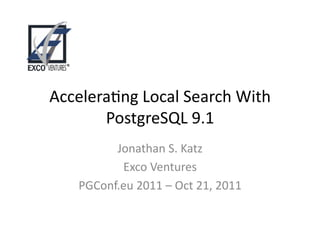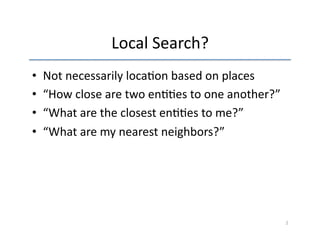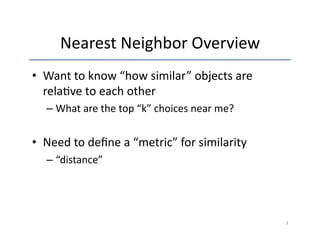Successfully reported this slideshow.

# Accelerating Local Search with PostgreSQL (KNN-Search)

10

Share×
1 of 26
1 of 26

# Accelerating Local Search with PostgreSQL (KNN-Search)

10

Share

KNN-GiST indexes were added in PostgreSQL 9.1 and greatly accelerate some common queries in the geospatial and textual search realms. This presentation will demonstrate the power of KNN-GiST indexes on geospatial and text searching queries, but also their present limitations through some of my experimentations. I will also discuss some of the theory behind KNN (k-nearest neighbor) as well as some of the applications this feature can be applied too.

To see a version of the talk given at PostgresOpen 2011, please visit http://www.youtube.com/watch?v=N-MD08QqGEM

KNN-GiST indexes were added in PostgreSQL 9.1 and greatly accelerate some common queries in the geospatial and textual search realms. This presentation will demonstrate the power of KNN-GiST indexes on geospatial and text searching queries, but also their present limitations through some of my experimentations. I will also discuss some of the theory behind KNN (k-nearest neighbor) as well as some of the applications this feature can be applied too.

To see a version of the talk given at PostgresOpen 2011, please visit http://www.youtube.com/watch?v=N-MD08QqGEM

## More Related Content

### Related Books

Free with a 14 day trial from Scribd

See all

### Related Audiobooks

Free with a 14 day trial from Scribd

See all

### Accelerating Local Search with PostgreSQL (KNN-Search)

1. 1. Accelera'ng Local Search With PostgreSQL 9.1 Jonathan S. Katz Exco Ventures PGConf.eu 2011 – Oct 21, 2011
2. 2. Local Search? • Not necessarily loca'on based on places • “How close are two en''es to one another?” • “What are the closest en''es to me?” • “What are my nearest neighbors?” 2
3. 3. Nearest Neighbor Overview • Want to know “how similar” objects are rela've to each other – What are the top “k” choices near me? • Need to define a “metric” for similarity – “distance” 3
4. 4. K-­‐Nearest Neighbor • Given a collec'on of n objects • When trying to classify an unknown object – compute the distance between all known objects – find the k (k ≥ 1) closest objects to the unknown object – classify the object based on class of k closest objects • When k=1, then unknown object is given same classifica'on as object it is closest to 4
5. 5. K=1 Example Voronoi Diagram of order 1 can be used to make k=1 NN queries 5
6. 6. Just a bit more theory… • Voronoi diagrams of order-­‐1 are created in O(n log n) 'me – Looks similar to…? :-­‐) • Queried in O(1) 'me • Therefore: – Pay the 'me penalty to build the index – Query against index quickly 6
7. 7. Applica'ons • Geoloca'on + Op'mizing Posi'oning • Classifica'on • Similarity • Recommenda'on systems • Content-­‐based image retrieval • etc. 7
8. 8. So what about PostgreSQL? • As of PostgreSQL 9.0 – supports geometric types and distances • Points, circles, lines, boxes, polygons • Distance operator: <-­‐> – pg_trgm – supplied module for determining text similarity • similarity(“abc”, “ade”) computes similarity score • <-­‐> defines distance (opposite if similarity), not defined (in 9.0) 8
9. 9. PostgreSQL 9.1: KNN-­‐GiST • Let n = size of a table • Can index data that provides a “<-­‐>” (distance) operator – Geometric – pg_trgm • “k” = LIMIT clause • Known inefficiencies when k=n and n is small 9
10. 10. Example: pg_trgm • Data: – List of 1,000,000 names – 700,000 unique – n = 1,000,000 • Indexes: – CREATE INDEX names_name_idx ON names (name) – CREATE INDEX trgm_idx ON names USING gist (name gist_trgm_ops) • k=10 • Displaying query plan / execu'on 'me aqer 10 runs 10
11. 11. pg_trgm: 9.0 EXPLAIN ANALYZE SELECT name, similarity(name, 'jon') AS sim FROM names WHERE name % 'jon' ORDER BY sim DESC LIMIT 10; 11
12. 12. pg_trgm: 9.0 Limit (cost=2724.95..2724.98 rows=10 width=14) (actual 'me=192.793..192.794 rows=10 loops=1) -­‐> Sort (cost=2724.95..2727.45 rows=1000 width=14) (actual 'me=192.790..192.791 rows=10 loops=1) Sort Key: (similarity(name, 'jon'::text)) Sort Method: top-­‐N heapsort Memory: 25kB -­‐> Bitmap Heap Scan on names (cost=56.47..2703.34 rows=1000 width=14) (actual 'me=188.836..192.499 rows=865 loops=1) Recheck Cond: (name % 'jon'::text) -­‐> Bitmap Index Scan on trgm_idx (cost=0.00..56.22 rows=1000 width=0) (actual 'me=188.652..188.652 rows=865 loops=1) Index Cond: (name % 'jon'::text) Total run'me: 192.881 ms 12
13. 13. pg_trgm: 9.1 EXPLAIN ANALYZE SELECT name, similarity(name, 'jon') AS sim FROM names WHERE name % 'jon' ORDER BY sim DESC LIMIT 10; 13
14. 14. pg_trgm 9.1 Limit (cost=2720.91..2720.93 rows=10 width=14) (actual 'me=202.022..202.023 rows=10 loops=1) -­‐> Sort (cost=2720.91..2723.41 rows=1000 width=14) (actual 'me=202.020..202.021 rows=10 loops=1) Sort Key: (similarity(name, 'jon'::text)) Sort Method: top-­‐N heapsort Memory: 25kB -­‐> Bitmap Heap Scan on names (cost=52.43..2699.30 rows=1000 width=14) (actual 'me=198.324..201.719 rows=865 loops=1) Recheck Cond: (name % 'jon'::text) -­‐> Bitmap Index Scan on names_trgm_idx (cost=0.00..52.18 rows=1000 width=0) (actual 'me=198.156..198.156 rows=865 loops=1) Index Cond: (name % 'jon'::text) Total run1me: 202.113 ms 14
15. 15. Comparable? • Seems to be similar – Need to do more research why – anyone? • However, 9.1 offers improvements for LIKE/ ILIKE search with pg_trgm 15
16. 16. LIKE/ILIKE EXPLAIN ANALYZE SELECT name FROM names WHERE name LIKE '%ata%n'; 16
17. 17. LIKE/ILIKE pg_trgm: 9.0 vs 9.1 Seq Scan on names (cost=0.00..18717.00 rows=99 width=14) (actual 'me=0.339..205.659 rows=665 loops=1) Filter: (name ~~ '%ata%n'::text) Total run1me: 205.743 ms Bitmap Heap Scan on names (cost=9.45..369.20 rows=99 width=14) (actual 'me=122.494..125.967 rows=665 loops=1) Recheck Cond: (name ~~ '%ata%n'::text) -­‐> Bitmap Index Scan on names_trgm_idx (cost=0.00..9.42 rows=99 width=0) (actual 'me=121.972..121.972 rows=3551 loops=1) Index Cond: (name ~~ '%ata%n'::text) Total run1me: 126.065 ms 17
18. 18. Geometry • Data: – 2,000,000 points, from (0,0) -­‐> (10000, 10000) • Index: – CREATE INDEX geoloc_coord_idx ON geoloc USING gist (coord); 18
19. 19. Geometry EXPLAIN ANALYZE SELECT *, coord <-­‐> point(500,500) FROM geoloc ORDER BY coord <-­‐> point(500,500) LIMIT 10; 19
20. 20. Geometry: 9.0 vs 9.1 Limit (cost=80958.28..80958.31 rows=10 width=20) (actual 'me=1035.313..1035.316 rows=10 loops=1) -­‐> Sort (cost=80958.28..85958.28 rows=2000000 width=20) (actual 'me=1035.312..1035.314 rows=10 loops=1) Sort Key: ((coord <-­‐> '(500,500)'::point)) Sort Method: top-­‐N heapsort Memory: 25kB -­‐> Seq Scan on geoloc (cost=0.00..37739.00 rows=2000000 width=20) (actual 'me=0.029..569.501 rows=2000000 loops=1) Total run1me: 1035.349 ms Limit (cost=0.00..0.81 rows=10 width=20) (actual 'me=0.576..1.255 rows=10 loops=1) -­‐> Index Scan using geoloc_coord_idx on geoloc (cost=0.00..162068.96 rows=2000000 width=20) (actual 'me=0.575..1.251 rows=10 loops=1) Order By: (coord <-­‐> '(500,500)'::point) Total run1me: 1.391 ms 20
21. 21. Applica'on Examples • Proximity map search – fast! 21
22. 22. Drawbacks • Performance benefits are limited when: – LIMIT is close to size of data set and data set is large – Data set is small • Time to build index – High transac'on table 22
23. 23. Conclusions • GiST: “Generalized Search Tree” – index is there, up to developers to define access methods of data types – e.g. yields KNN-­‐GiST • Different types of applica'ons can be built – performance enhancements • Next steps? 23
24. 24. My Wish List • Further geometric-­‐type support in Postgres – N-­‐dimensional points – ‘=‘ operator for point type – (PostGIS s'll champion of complex geometric + geographic data types) • Define “distance” over mul'columns with different types? – SELECT (a.name, a.geocode) <-­‐> (b.name, b.geocode) FROM x a, x b; 24
25. 25. References • Oleg Bartunov – “Efficient K-­‐nearest neighbour search in PostgreSQL” (h~p://www.sai.msu.su/~megera/ postgres/talks/pgday-­‐2010.pdf) • Oleg Bartunov and Teodor Sigaev for work on KNN-­‐ GiST and notes on pg_trgm (h~p:// developer.postgresql.org/pgdocs/postgres/ pgtrgm.html) • Hubert “depesz” Lubaczewski – pa~erned benchmarks off of his work -­‐ h~p://www.depesz.com/index.php/ 2010/12/11/wai'ng-­‐for-­‐9-­‐1-­‐knngist/ 25
26. 26. Contact • jonathan.katz@excoventures.com • @jkatz05 • Feedback Please! – h~p://2011.pgconf.eu/feedback 26